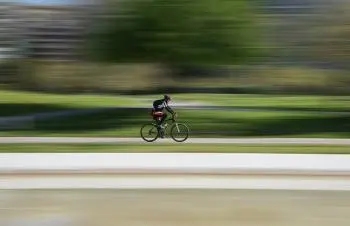# Linear Velocity: Formulas, Definition, Examples and ExercisesLinear velocity is a fundamental concept in physics used to describe how fast an object moves along a straight-line path. This magnitude is of great importance in daily life and in various scientific disciplines such as physics, engineering and mechanics.

In this article, we will explain in detail what linear velocity is, how it is calculated, provide examples of its application in daily life and solve some practical exercises.

## Definition of Linear VelocityLinear velocity, often denoted as "v," is a measure of how fast an object is moving along a straight path. It is defined as the distance traveled by the object divided by the time that has elapsed.

In the International System (SI), the fundamental unit for measuring linear speed is the meter per second (m/s). However, in everyday life, more familiar units are used, such as the kilometer per hour (km/h), which is commonly used to measure the speed of land vehicles such as cars, bicycles, and trains.

## Formulas

Mathematically, linear velocity is expressed as follows:

v=d/t

Where:

• v is the linear velocity in units of length per unit of time (e.g., meters per second, kilometers per hour).

• d is the distance traveled by the object in units of length (e.g., meters, kilometers).

• t is the elapsed time in time units (e.g., seconds, hours).

However, there are several more specific ways to calculate linear velocity depending on the information available. Here are some common formulas:

### Average Linear Speed

When the total distance traveled (d) and the total time (t) are known, the average linear speed is calculated as:

v average =d/t

### Instant Speed

Instantaneous speed refers to the speed at a specific point in time. To calculate it, you need the displacement (Δd) and the change in time (Δt):

v instantaneous =Δd / Δt

### Relative Speed

When dealing with two moving objects, the relative velocity is calculated by subtracting the velocities of the two objects:

relative v =v 1 −v 2

Where v1 is the speed of the first object and v2 is the speed of the second object.

## Types of Movements That Include Linear Velocity

Linear velocity applies to various types of motion in which an object moves in a straight line.

Here are some examples of types of movements that involve linear velocity:

### Uniform Rectilinear Motion (MRU)

In uniform rectilinear motion, an object moves in a straight line at a constant speed. The linear speed in an MRU is constant, and the distance traveled is directly proportional to the elapsed time.

Examples include a car traveling on a highway at a constant speed or a person walking at a constant pace.

### Uniformly Accelerated Rectilinear Motion (MRUA)

In uniformly accelerated rectilinear motion, an object moves in a straight line, but its speed changes uniformly with time due to a constant acceleration. Linear velocity increases or decreases at a constant rate.

Examples include a car gradually braking or an object falling due to gravity.

### Parabolic ShotWhen an object is launched into the air and follows a parabolic path, its horizontal motion is an MRU, while its vertical motion may be an MRUA due to gravity.

The linear velocity of the parabolic shot is used to describe how fast it travels horizontally.

## Examples in Everyday Life

Linear velocity is found in numerous aspects of our daily lives. Here are some examples:

• Car Driving : When you drive a car, you are constantly calculating and adjusting your linear speed to maintain a safe speed and reach your destination on time. If you travel at a speed of 60 kilometers per hour for 2 hours, you will have traveled 120 kilometers.
• Walk : When you walk, your linear speed is much lower than that of a car, but it is still important. If you walk at a speed of 5 kilometers per hour for 30 minutes, you will have traveled 2.5 kilometers.
• Cycling : Cyclists also use linear speed to measure their performance and plan routes. If you pedal at a constant speed of 20 kilometers per hour for 1.5 hours, you will have traveled 30 kilometers.

## Solved Exercises

Now, let's solve some practical exercises related to linear velocity:

### Exercise 1

A car travels a distance of 400 kilometers in a time of 4 hours. What is the average linear speed of the car?

Solution

We use the average linear velocity formula:

v average =d/t=400 km / 4 h=100 km/h

The average linear speed of the car is 100 kilometers per hour.

### Exercise 2

A runner completes a 10 kilometer race in a time of 45 minutes. Calculate its average linear speed in meters per second.

Solution

First, we convert the time to seconds, since 1 minute is equal to 60 seconds:

t=45 minutes=45×60 seconds=2700 seconds

Now we use the average linear velocity formula:

v average =d/t=10 km / 2700 s =10000 m / 2700 s≈3.7 m/s

The runner's average linear speed is approximately 3.7 meters per second.

### Exercise 3Two trains are moving in opposite directions. The first train has a speed of 80 km/h, and the second train has a speed of 100 km/h. What is the relative speed between the two trains?

Solution

We use the relative speed formula:

relative v =v 1 −v 2 =80 km/h−100 km/h=−20 km/h

The relative speed between the two trains is 20 kilometers per hour in opposite directions. The negative sign indicates that they are moving in opposite directions.

Author:

Published: September 21, 2023
Last review: September 21, 2023